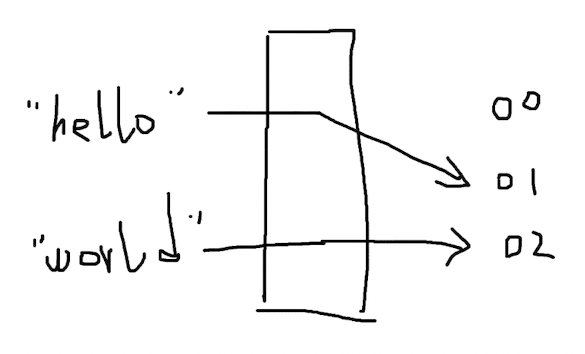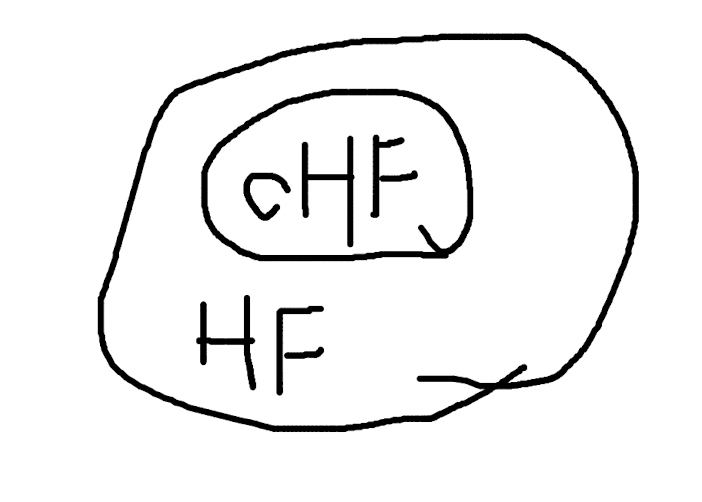# Hash function

## Hash function

Hash function 用于处理数据和其 hash values 的映射关系，hash values 是数据类似唯一标识的东西，可以用内存比较小的形式标识数据。hash function 有各种各样的实现，可以认为是一个黑盒子，进去的是 data，出来的是 hash values。``````HASH("a") = 97
HASH("b") = 98
HASH("c") = 99
HASH("d") = 100
``````

``````HASH("ab") = 97 + 98 = 195
HASH("cd") = 99 + 100 = 199
``````

hash values 是允许重复的，但如果存在大量重复，hash function 也就失去了它的作用和使用场景：如果全部都一样，无法区分，还用 hash values 干嘛？

``````HASH("ab") = 97 * 1000 + 98 = 97098
HASH("cd") = 99 * 1000 + 100 = 99100
HASH("ad") = 97 * 1000 + 100 = 97100
HASH("cd") = 98 * 1000 + 99 = 98099
``````

``````hashCodes = char1 * base^(l-1) + char2 * base^(l-2) + ...
``````

`hashCodes` 是输出的 hash values，`char1` 是第 1 个字符，`char2` 是第 2个字符，`base` 是基数，`l` 指字符串的长度。对于 3 个字符长度的字符串，第 1 个字符的基数就是质数的 2 次方，第 2 个字符的基数是质数的 1 次方，第 3 个字符是 0 次方，以此类推。

``````public static int hashCode(byte[] value) {
int h = 0;
for(int i = 0; i < value.length; ++i) {
h = 31 * h + value[i];
}
return h;
}
``````

``````hashCode("a") = 97
hashCode("ab") = 97 * 31 + 98 = 3105
hashCode("abc") = 97*31^2 + 98*31 + 99 = 96354
``````

## Cryptographic hash function (CHF)

`````` public static String deHashCode(int code) {
for (int x = 0; x <= 255; x++) {
int y = code - 31 * x;
if (y < 0 || y > 255) {
continue;
}
System.out.println(((char) x)+","+((char) y));
}
return "";
}
``````

``````\,ý
],Þ
^,¿
_,
`,
a,b
b,C
c,\$
d,
``````

cryptographic 是 hash function 的修饰词，即使用了加密算法的 hash function。md5 是使用非常广泛也接近过时的一种 cryptographic hash function，可以把任意长度的 data 计算输出为 128 bit 的 hash values。

``````md5("a") = 0cc175b9c0f1b6a831c399e269772661
md5("ab") = 187ef4436122d1cc2f40dc2b92f0eba0
``````

md5 的加密原理步骤很多，是一种不可逆的、单向的 hash function，无法轻易根据 hash values 得到 data。md5 的输入可以是任意大小的，1 GB 的二进制文件也可以hash 为 128 bit 的字符串。

md5 之外，SHA-1 的安全性更高，BLAKE2 的计算速度更快，它们都是典型的 cryptographic hash function。